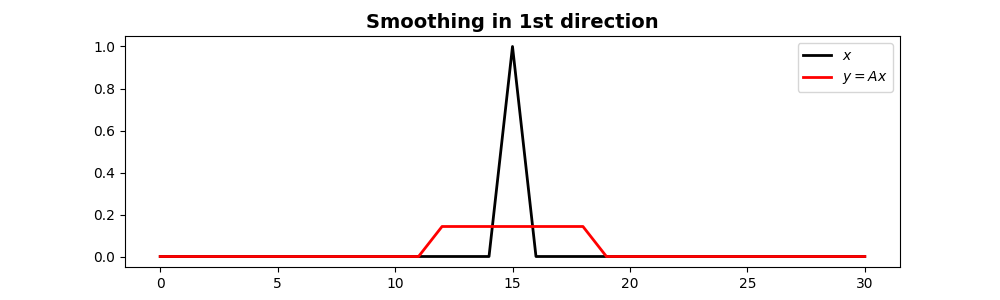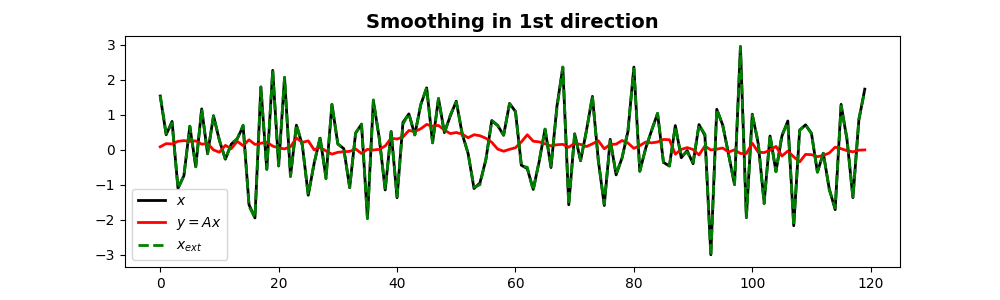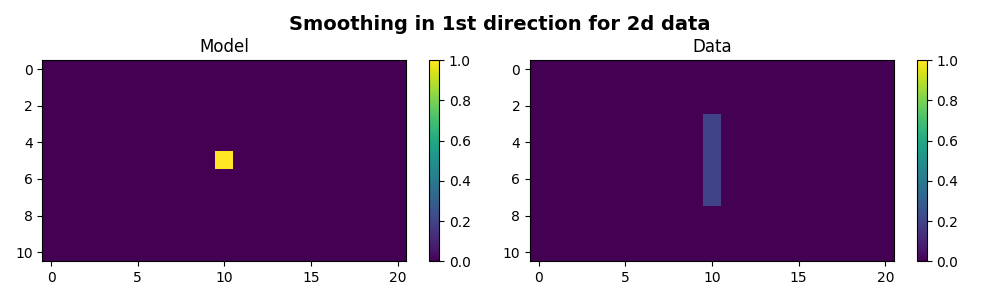# 1D Smoothing¶

This example shows how to use the pylops.Smoothing1D operator to smooth an input signal along a given axis.

Derivative (or roughening) operators are generally used regularization in inverse problems. Smoothing has the opposite effect of roughening and it can be employed as preconditioning in inverse problems.

A smoothing operator is a simple compact filter on lenght $$n_{smooth}$$ and each elements is equal to $$1/n_{smooth}$$.

import matplotlib.pyplot as plt
import numpy as np

import pylops

plt.close("all")


Define the input parameters: number of samples of input signal (N) and lenght of the smoothing filter regression coefficients ($$n_{smooth}$$). In this first case the input signal is one at the center and zero elsewhere.

N = 31
nsmooth = 7
x = np.zeros(N)
x[int(N / 2)] = 1

Sop = pylops.Smoothing1D(nsmooth=nsmooth, dims=[N], dtype="float32")

y = Sop * x
xadj = Sop.H * y

fig, ax = plt.subplots(1, 1, figsize=(10, 3))
ax.plot(x, "k", lw=2, label=r"$x$")
ax.plot(y, "r", lw=2, label=r"$y=Ax$")
ax.set_title("Smoothing in 1st direction", fontsize=14, fontweight="bold")
ax.legend()Out:

<matplotlib.legend.Legend object at 0x7f4df588b9e8>


Let’s repeat the same exercise with a random signal as input. After applying smoothing, we will also try to invert it.

N = 120
nsmooth = 13
x = np.random.normal(0, 1, N)
Sop = pylops.Smoothing1D(nsmooth=13, dims=(N), dtype="float32")

y = Sop * x
xest = Sop / y

fig, ax = plt.subplots(1, 1, figsize=(10, 3))
ax.plot(x, "k", lw=2, label=r"$x$")
ax.plot(y, "r", lw=2, label=r"$y=Ax$")
ax.plot(xest, "--g", lw=2, label=r"$x_{ext}$")
ax.set_title("Smoothing in 1st direction", fontsize=14, fontweight="bold")
ax.legend()Out:

<matplotlib.legend.Legend object at 0x7f4df5744710>


Finally we show that the same operator can be applied to multi-dimensional data along a chosen axis.

A = np.zeros((11, 21))
A[5, 10] = 1

Sop = pylops.Smoothing1D(nsmooth=5, dims=(11, 21), dir=0, dtype="float64")
B = np.reshape(Sop * np.ndarray.flatten(A), (11, 21))

fig, axs = plt.subplots(1, 2, figsize=(10, 3))
fig.suptitle(
"Smoothing in 1st direction for 2d data", fontsize=14, fontweight="bold", y=0.95
)
im = axs.imshow(A, interpolation="nearest", vmin=0, vmax=1)
axs.axis("tight")
axs.set_title("Model")
plt.colorbar(im, ax=axs)
im = axs.imshow(B, interpolation="nearest", vmin=0, vmax=1)
axs.axis("tight")
axs.set_title("Data")
plt.colorbar(im, ax=axs)
plt.tight_layout()